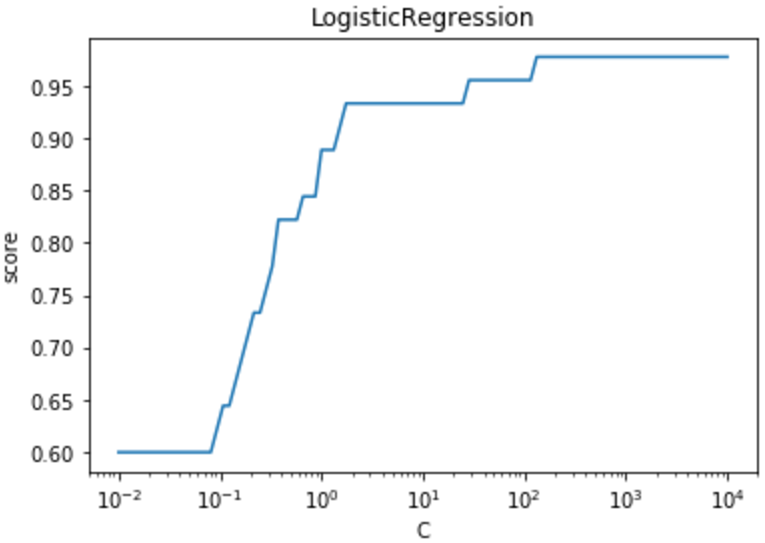机器学习教程之3-逻辑回归(logistic regression)的sklearn实现

2.假说表示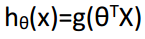S形函数(Sigmoid function):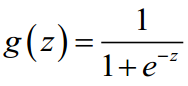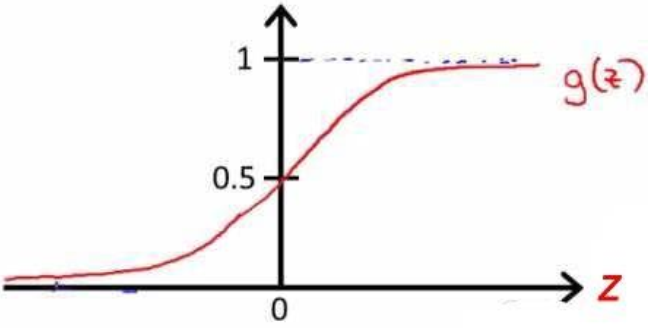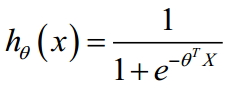hθ(x)的作用是，对于给定的输入变量，根据选择的参数计算输出变量=1 的可能性（estimated probablity）即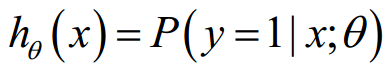4.代价函数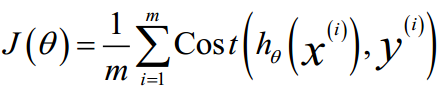，其中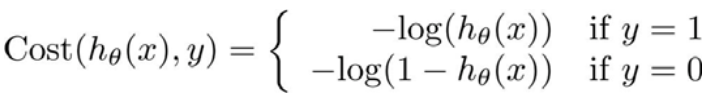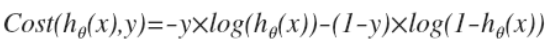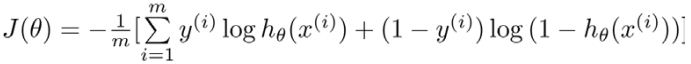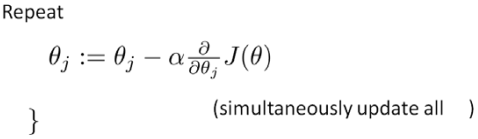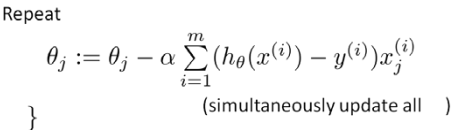5.简化的代价函数和梯度下降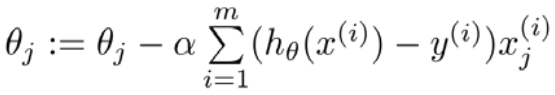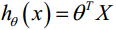8.代码

(1)原始模型

"""

"""

"""

"""
import numpy as np
import matplotlib.pyplot as plt

# 使用交叉验证的方法，把数据集分为训练集合测试集
from sklearn.model_selection import train_test_split

from sklearn import datasets
from sklearn.linear_model import LogisticRegression

# 加载iris数据集

# 将数据集拆分为训练集和测试集
X_train, X_test, y_train, y_test = train_test_split(
diabetes.data, diabetes.target, test_size=0.30, random_state=0)
return X_train, X_test, y_train, y_test

# 使用LogisticRegression考察线性回归的预测能力
def test_LogisticRegression(X_train, X_test, y_train, y_test):
# 选择模型
cls = LogisticRegression()

# 把数据交给模型训练
cls.fit(X_train, y_train)

print("Coefficients:%s, intercept %s"%(cls.coef_,cls.intercept_))
print("Residual sum of squares: %.2f"% np.mean((cls.predict(X_test) - y_test) ** 2))
print('Score: %.2f' % cls.score(X_test, y_test))

if __name__=='__main__':
test_LogisticRegression(X_train,X_test,y_train,y_test) # 调用 test_LinearRegression

Coefficients:[[ 0.40051422 1.30952762 -2.09555215 -0.9602869 ]
[ 0.3779536 -1.39504236 0.41399108 -1.09189364]
[-1.66918252 -1.18193972 2.39506569 2.00963954]], intercept [ 0.24918551 0.81149187 -0.97217565]
Residual sum of squares: 0.11
Score: 0.89

(2)在(1)的基础上使用多分类参数

"""

"""

"""

"""
import numpy as np
import matplotlib.pyplot as plt

# 使用交叉验证的方法，把数据集分为训练集合测试集
from sklearn.model_selection import train_test_split

from sklearn import datasets
from sklearn.linear_model import LogisticRegression

# 加载iris数据集

# 将数据集拆分为训练集和测试集
X_train, X_test, y_train, y_test = train_test_split(
diabetes.data, diabetes.target, test_size=0.30, random_state=0)
return X_train, X_test, y_train, y_test

# 使用LogisticRegression考察线性回归的预测能力
def test_LogisticRegression_multiomaial(X_train, X_test, y_train, y_test):
# 选择模型
cls = LogisticRegression(multi_class='multinomial',solver='lbfgs')

# 把数据交给模型训练
cls.fit(X_train, y_train)

print("Coefficients:%s, intercept %s"%(cls.coef_,cls.intercept_))
print("Residual sum of squares: %.2f"% np.mean((cls.predict(X_test) - y_test) ** 2))
print('Score: %.2f' % cls.score(X_test, y_test))

if __name__=='__main__':
test_LogisticRegression_multiomaial(X_train,X_test,y_train,y_test) # 调用 test_LinearRegression

Coefficients:[[-0.39772352 0.83347392 -2.28853669 -0.98142875]
[ 0.54455173 -0.29022825 -0.23370111 -0.65566222]
[-0.14682821 -0.54324567 2.5222378 1.63709097]], intercept [ 8.99974988 1.54361012 -10.54336001]
Residual sum of squares: 0.02
Score: 0.98

(3)考虑正则化系数

"""

"""

"""

"""
import numpy as np
import matplotlib.pyplot as plt

# 使用交叉验证的方法，把数据集分为训练集合测试集
from sklearn.model_selection import train_test_split

from sklearn import datasets
from sklearn.linear_model import LogisticRegression

# 加载iris数据集

# 将数据集拆分为训练集和测试集
X_train, X_test, y_train, y_test = train_test_split(
diabetes.data, diabetes.target, test_size=0.30, random_state=0)
return X_train, X_test, y_train, y_test

# 使用LogisticRegression考察线性回归的预测能力
def test_LogisticRegression_C(X_train, X_test, y_train, y_test):
Cs=np.logspace(-2,4,num=100)
scores=[]
for C in Cs:
# 选择模型
cls = LogisticRegression(C=C)

# 把数据交给模型训练
cls.fit(X_train, y_train)

scores.append(cls.score(X_test, y_test))

## 绘图
fig=plt.figure()
ax.plot(Cs,scores)
ax.set_xlabel(r"C")
ax.set_ylabel(r"score")
ax.set_xscale('log')
ax.set_title("LogisticRegression")
plt.show()

if __name__=='__main__':
test_LogisticRegression_C(X_train,X_test,y_train,y_test) # 调用 test_LinearRegression